#DAY 22
1
Software Development

## Packet-Analytics with Data Science

Motivation:

• 想知道 PCAP 檔(封包檔) 藏了什麽祕密
• 延伸: 分析流量 以二元區分: 來亂 or 正常 , 多元區分: DDoS , DoS , Scan , Theft ... 等等
• 知己(方法待定)知彼(PCAP) 百戰百勝: 如果知道大致的差異,就用簡單的方式大致分類,看不出差異再用特定分析方法: Anova? 時間序列？

Scapy Output -> Pandas DataFrame --> Visualizations

• Visualizations
3. Source Ports (Bytes Sent)
5. History of bytes sent by most frequent address
• Result: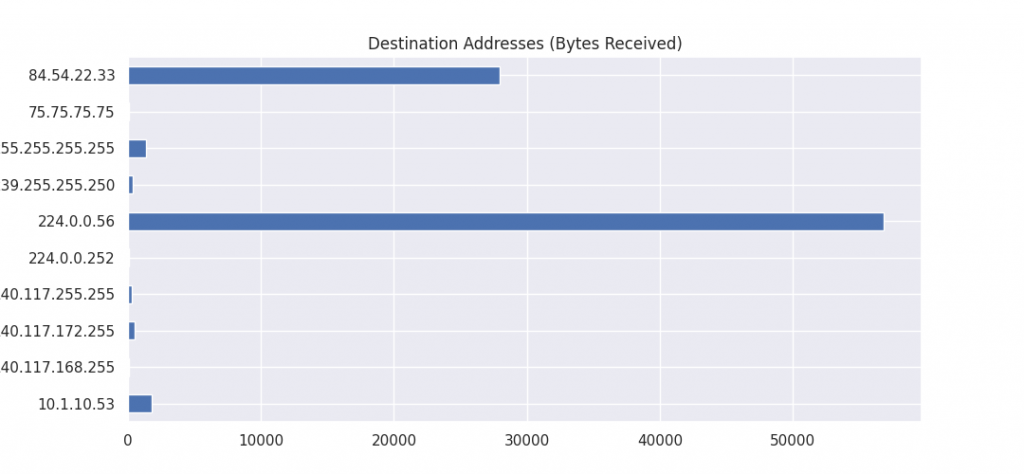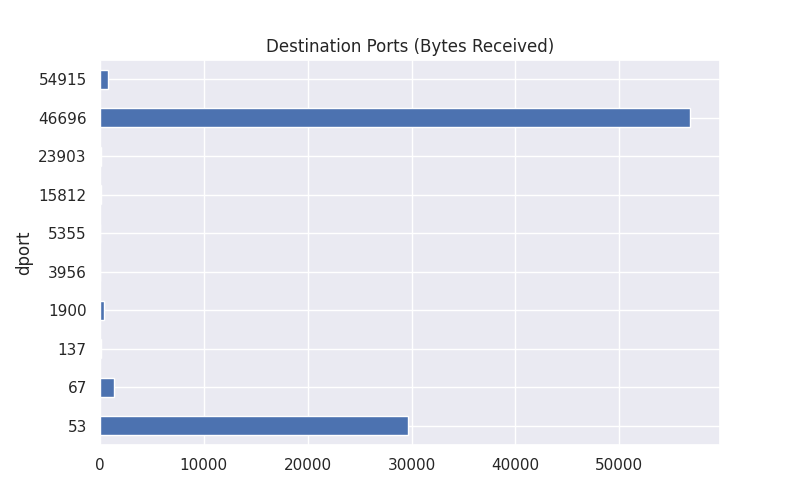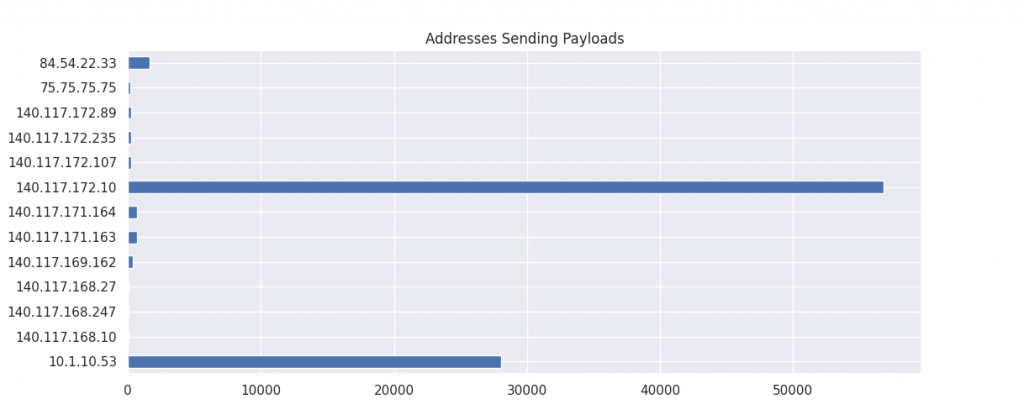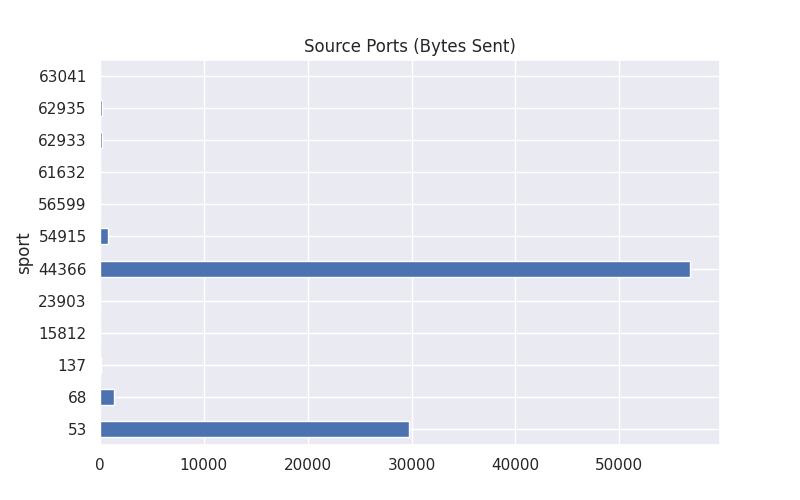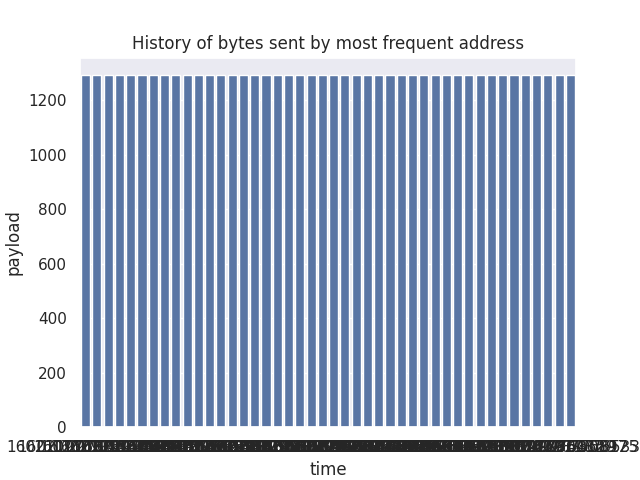Learning Packet Analysis with Data Science

No Jupyter But Only Terminal env. Version:
PS 1: 自己改寫版,有刪除部份程式碼,只留下輸出圖片的部份
PS 2: suspicious.pcap 可在 這裡 取得

\$ sudo python3 packetAnalytics.py

``````from scapy.all import * # Packet manipulation
import pandas as pd # Pandas - Create and Manipulate DataFrames
import numpy as np # Math Stuff (don't worry only used for one line :] )
import binascii # Binary to Ascii
import seaborn as sns
sns.set(color_codes=True)

'''Use common fields in IP Packet to perform exploratory analysis on PCAP'''

num_of_packets_to_sniff = 100
pcap = sniff(count=num_of_packets_to_sniff)
pcap = pcap+rdpcap("suspicious.pcap")

# Scapy provides this via import statements
from scapy.layers.l2 import Ether
from scapy.layers.inet import IP
from scapy.layers.inet import TCP, UDP

# Collect field names from IP/TCP/UDP (These will be columns in DF)
ip_fields = [field.name for field in IP().fields_desc]
tcp_fields = [field.name for field in TCP().fields_desc]
udp_fields = [field.name for field in UDP().fields_desc]

# Create blank DataFrame
df = pd.DataFrame(columns=dataframe_fields)
for packet in pcap[IP]:
# Field array for each row of DataFrame
field_values = []
# Add all IP fields to dataframe
for field in ip_fields:
if field == 'options':
# Retrieving number of options defined in IP Header
field_values.append(len(packet[IP].fields[field]))
else:
field_values.append(packet[IP].fields[field])

field_values.append(packet.time)

for field in tcp_fields:
try:
if field == 'options':
field_values.append(len(packet[layer_type].fields[field]))
else:
field_values.append(packet[layer_type].fields[field])
except:
field_values.append(None)

df_append = pd.DataFrame([field_values], columns=dataframe_fields)
df = pd.concat([df, df_append], axis=0)

# Reset Index
df = df.reset_index()
# Drop old index column
df = df.drop(columns="index")

import matplotlib.pyplot as plt

plt.show()

# Group by Source Port and Payload Sum
plt.show()

label="Total", color="b").set_title("History of bytes sent by most frequent address")
plt.show()

plt.show()

plt.show()

plt.show()

# Create dataframe with only converation from most frequent address

plt.show()

'''
# Which address has excahnged the most amount of bytes with most frequent address